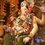# Trigonometry with the Ancient Indian Mathematicians

The most significant development of trigonometry in ancient times was in India. Influential works from the $4^{th}$ & the $5^{th}$ century AD, known as Siddhantas first defined the sine as the relationship between half an angle & half a chord, while also defining the cosine, versine ($1-$ cosine) & inverse sine.

Soon afterwards, another Indian mathematician & astronomer Aryabhata collected & expanded upon the developments of the Siddhantas in a path breaking work, the Aryabhatiya. The Siddhantas & the Aryabhatiya contain the earliest survivng tables of sine & versine values, in $3.75^\circ$ intervals from $0^\circ$ to $90^\circ$, to an accuracy of $4$ decimal places. They used the words jya for sine, kojya for cosine, utkrama-jya for versine & otkram jya for inverse sine. The words jya & kojya eventually became sine & cosine after a mistranslation.

Aryabhata was the first one. Others after him expanded on these works of trigonometry. In the $6^{th}$ century AD, Varahamihira discovered the identity $sin^2x + cos^2x = 1$. He improved Aryabhata's sine table & discovered an early version of the Pascal's Triangle.

Such was the ingenuity of the ancient Indian mathematicians!Note by Ameya Salankar
7 years, 3 months ago

This discussion board is a place to discuss our Daily Challenges and the math and science related to those challenges. Explanations are more than just a solution — they should explain the steps and thinking strategies that you used to obtain the solution. Comments should further the discussion of math and science.

When posting on Brilliant:

• Use the emojis to react to an explanation, whether you're congratulating a job well done , or just really confused .
• Ask specific questions about the challenge or the steps in somebody's explanation. Well-posed questions can add a lot to the discussion, but posting "I don't understand!" doesn't help anyone.
• Try to contribute something new to the discussion, whether it is an extension, generalization or other idea related to the challenge.

MarkdownAppears as
*italics* or _italics_ italics
**bold** or __bold__ bold
- bulleted- list
• bulleted
• list
1. numbered2. list
1. numbered
2. list
Note: you must add a full line of space before and after lists for them to show up correctly
paragraph 1paragraph 2

paragraph 1

paragraph 2

[example link](https://brilliant.org)example link
> This is a quote
This is a quote
    # I indented these lines
# 4 spaces, and now they show
# up as a code block.

print "hello world"
# I indented these lines
# 4 spaces, and now they show
# up as a code block.

print "hello world"
MathAppears as
Remember to wrap math in $$ ... $$ or $ ... $ to ensure proper formatting.
2 \times 3 $2 \times 3$
2^{34} $2^{34}$
a_{i-1} $a_{i-1}$
\frac{2}{3} $\frac{2}{3}$
\sqrt{2} $\sqrt{2}$
\sum_{i=1}^3 $\sum_{i=1}^3$
\sin \theta $\sin \theta$
\boxed{123} $\boxed{123}$

Sort by:

Great Indians great mathematics

- 5 years, 5 months ago